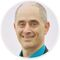cancel
Showing results for
Did you mean:
Collaborate forum
cancel
Showing results for
Did you mean:

## Elements schedule and total cost?Newcomer
All - I have an elements schedule with one field being QUANTITY. Another field is COST. Can someone tell me if it is possible to have a formula or expression to multiply QUANTITY times COST to get a total cost for the quantity?
When I try to do this expression, QUANTITY does not show as an available choice.
Thx
"Bob" - Church of the Sub-GeniusEnthusiast
Unfortunately this is not possible, since the Quantity you are referring to is derived from the number of elements grouped together in an Interactive Schedule, rather than a property or value associated with an individual element.

Expressions can calculate values based on the native properties of a specific element (e.g. length) in combination with other properties or constants (e.g. cost per length unit); thus one can derive the cost of a piece of steel based on the length times the cost per meter or foot. But this formula cannot refer to a quantity in a schedule, which is beyond the scope of the calculation.Latest solutions

Most liked posts